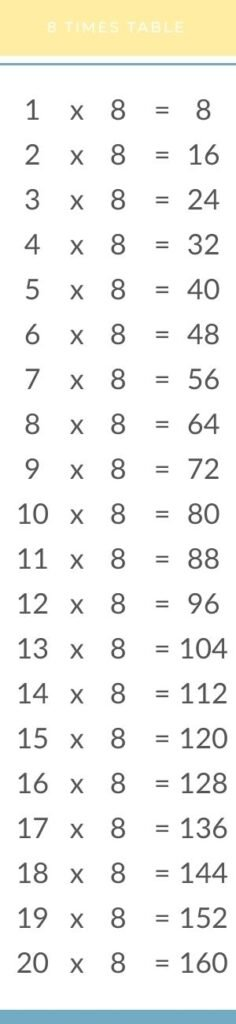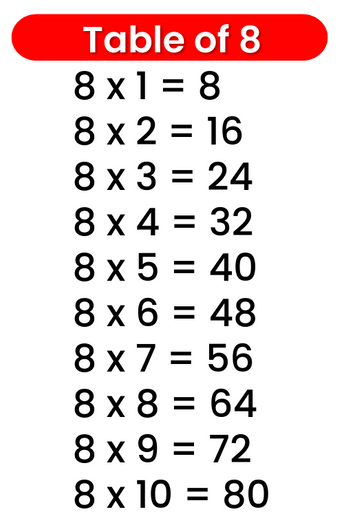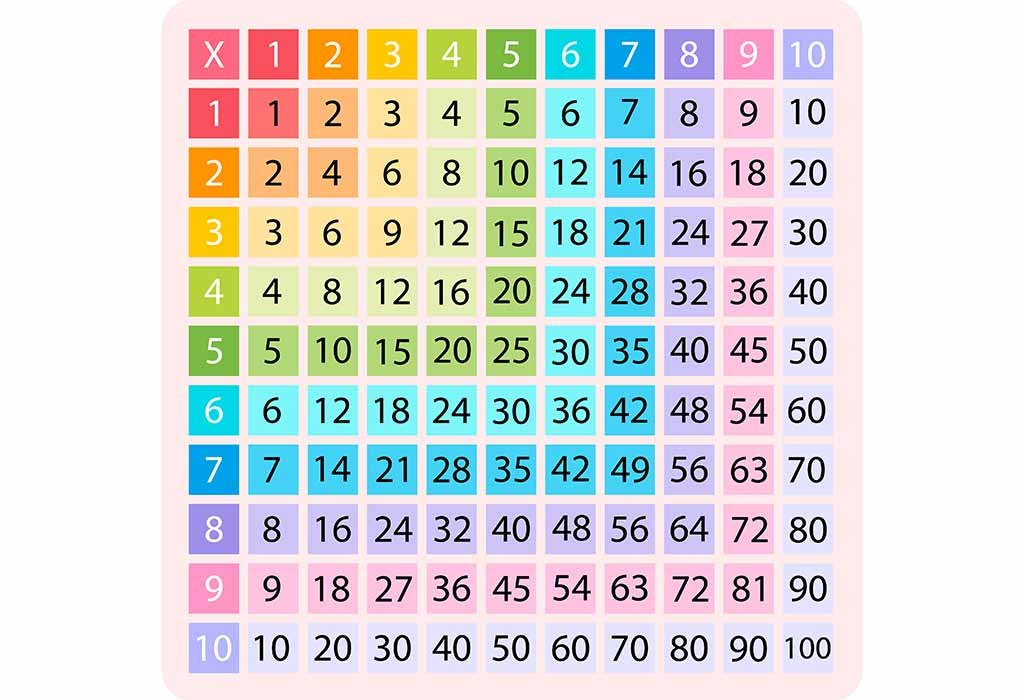# Times Table of 8, 8 ka table

## Times Table of 8, 8 ka table

Maths Multiplication Table of 8, Times Table of 8, 8 ka table, 8 ki Table Chart

8 × 1 = 8
8 × 2 = 16
8 × 3 = 24
8 × 4 = 32
8 × 5 = 40
8 × 6 = 48
8 × 7 = 56
8 × 8 = 64
8 × 9 = 72
8 × 10 = 80

## 8 ka table### Multiplication Tables of eight upto 20### Times Table of 8 chart upto 10